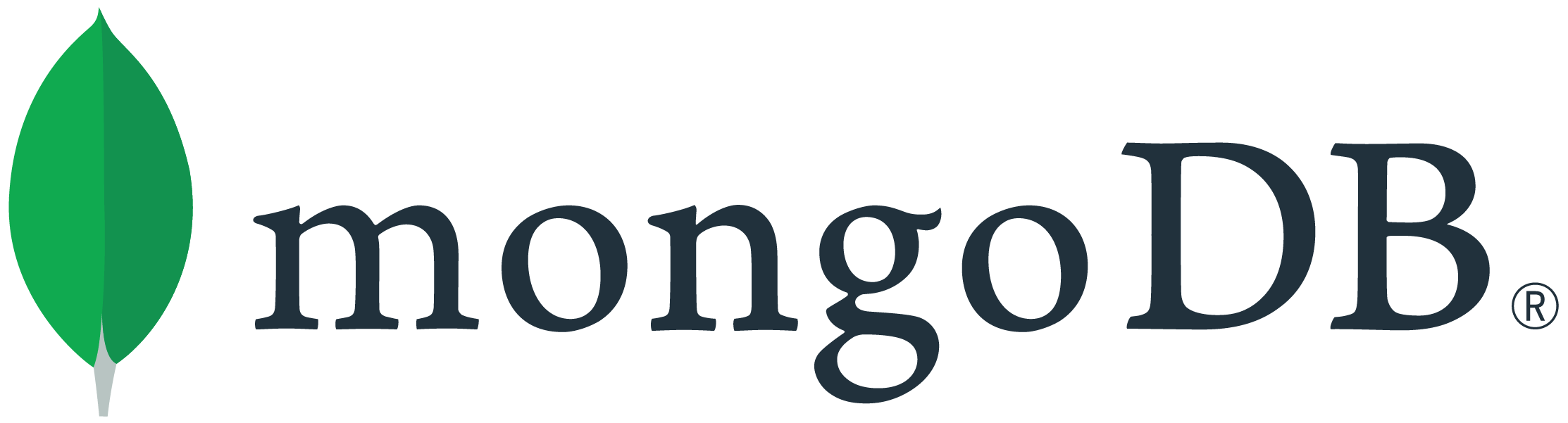# How to display the percentage of column total

Hello all,

In a stacked bar chart, I’ve customized the fields to display the percentage instead of decimal values. However it is displaying the percentage of grand total. But I need charts to display column wise percentage of subtotal.

Hi @Ashok_Rajan -

That percentage option is just number formatting - it won’t calculate the value as a percentage. We don’t have the ability to calculate each value as a percentage of the total yet, but you can request this at feedback.mongodb.com so we can consider it in the future.

Tom

2 Likes

Thank you @tomhollander

So to display the values as percentage should I create calculated fields for percentage and then move it to values?

Yes, you would need to precompute the percentage values using a pipeline or calculated fields.

Tom

1 Like

This topic was automatically closed 5 days after the last reply. New replies are no longer allowed.